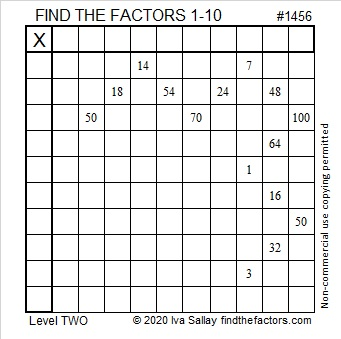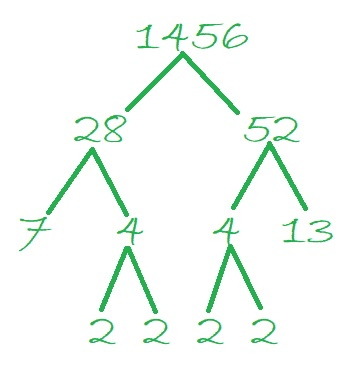# 1456 and Level 2

Contents

#### Today’s Puzzle:

Can you write each number from 1 to 10 in both the first column and the top row so that those numbers are the factors of the given clues?#### 1456 Factor Tree:

1456 is made from two multiples of 7, so we know it is divisible by 7. It’s last two digits are 56, so it is divisible by 4. Since it is divisible by 7 and by 4, it is divisible by 28. I used that fact to make this factor tree for 1456:#### Factors of 1456:

• 1456 is a composite number.
• Prime factorization: 1456 = 2 × 2 × 2 × 2 × 7 × 13, which can be written 1456 = 2⁴ × 7 × 13
• 1456 has at least one exponent greater than 1 in its prime factorization so √1456 can be simplified. Taking the factor pair from the factor pair table below with the largest square number factor, we get √1456 = (√16)(√91) = 4√91. The exponents in the prime factorization are 4, 1, and 1. Adding one to each exponent and multiplying we get (4 + 1)(1 + 1)(1 + 1) = 5 × 2 × 2 = 20. Therefore 1456 has exactly 20 factors.
• The factors of 1456 are outlined with their factor pair partners in the graphic below:#### Interesting facts about the number 1456:

1456 is the difference of two squares in six different ways:
365² – 363² = 1456
184² – 180² = 1456
95² – 87² = 1456
59² – 45² = 1456
41² – 15² = 1456
40² – 12² = 1456

1456 is the hypotenuse of one Pythagorean triple:
560-1344-1456 which is (5-12-13) times 112

This site uses Akismet to reduce spam. Learn how your comment data is processed.